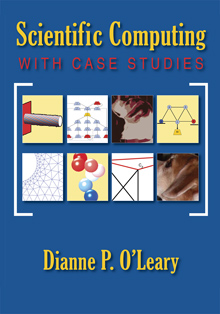# Scientific Computing with Case Studies

## by Dianne P. O'Leary

SIAM Press , 2009Learning through doing is the foundation of this book, which allows readers to explore case studies as well as expository material. The book provides a practical guide to the numerical solution of linear and nonlinear equations, differential equations, optimization problems, and eigenvalue problems. It treats standard problems and introduces important variants such as sparse systems, differential-algebraic equations, constrained optimization, Monte Carlo simulations, and parametric studies. Stability and error analysis is emphasized, and the MATLAB algorithms are grounded in sound principles of software design and in the understanding of machine arithmetic and memory management.

Nineteen case studies allow readers to become familiar with mathematical modeling and algorithm design, motivated by problems in physics, engineering, epidemiology, chemistry, and biology.

Course instructors: If this is a textbook for your course, contact Elizabeth Greenspan (greenspan at siam.org) to arrange for a 20% discount for your students (30% if your institution is an institutional member of SIAM).

This website contains supplementary material for the book.

• Index for the book
• Errata for the book

• Additional notes, exercises, slides, handouts, and case studies, provided without solutions.

• Solution Manual for challenges in the book (Version dated January 2009)
• Errata for the solution manual

• Data and sample Matlab programs for the challenges in the book. The programs are not perfect; in particular, the output is not always clearly explained, and the programs sometimes compute for a long time without warning the user that there will be a delay.

• Unit I. Preliminaries: Mathematical Modeling, Errors, Hardware and Software
• Sensitivity Analysis: When a Little Means a Lot
• Computer Memory and Arithmetic: A Look Under the Hood
• Design of Computer Programs: Writing Your Legacy
• Unit II. Dense Matrix Computations
• Case Study: Image Deblurring: I Can See Clearly Now
• Case Study: Updating and Downdating Matrix Factorizations: A Change in Plans
• Case Study: The Direction-of-Arrival Problem: Coming at You
• Unit III. Optimization and Data Fitting
• Case Study: Classified Information: The Data Clustering Problem
• Case Study: Achieving a Common Viewpoint: Yaw, Pitch, and Roll
• Case Study: Fitting Exponentials: An Interest in Rates
• Case Study: Blind Deconvolution: Errors, Errors Everywhere
• Case Study: Blind Deconvolution: A Matter of Norm
• Unit IV. Monte Carlo Computations
• Case Study: Monte-Carlo Minimization and Counting: One, Two, Too Many
• Case Study: Multidimensional Integration: Partition and Conquer
• Case Study: Models of Infection: Person to Person
• Unit V. Solution of Differential Equations
• Volterra predator/prey model for rabbits and foxes
• Case Study: More Models of Infection: It's Epidemic
• Case Study: Robot Control: Swinging Like a Pendulum
• Case Study: Finite Differences and Finite Elements: Getting to Know You
• Unit VI. Nonlinear Systems and Continuation Methods
• Case Study: Variable-Geometry Trusses
• Case Study: Beetles, Cannibalism, and Chaos
• Unit VII. Sparse Matrix Computations, with Applications to Partial Differential Equations
• Solving Sparse Linear Systems: Taking the Direct Approach
• Iterative Methods for Linear Systems
• Case Study: Elastoplastic Torsion: Twist and Stress
• Case Study: Fast Solvers and Sylvester Equations: Both Sides Now
• Case Study: Eigenvalues: Valuable Principles
• Multigrid Methods: Managing Massive Meshes
• Some of the partial solutions are revisions of articles originally printed in ``Computing in Science and Engineering," Copyright IEEE. These are posted here with permission of the IEEE. Internal or personal use of this material is permitted. However, permission to reprint/republish this material for advertising or promotional purposes or for creating new collective works for resale or redistribution must be obtained from the IEEE by writing to pubs-permissions@ieee.org.